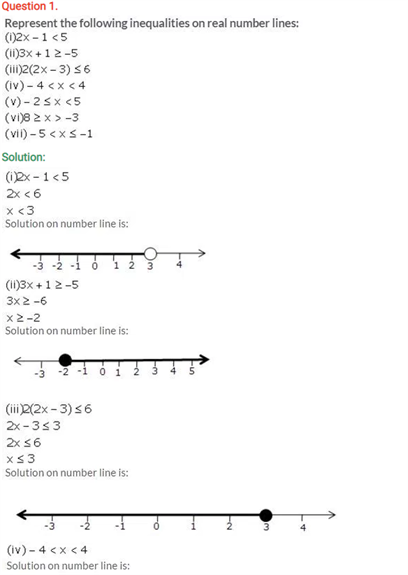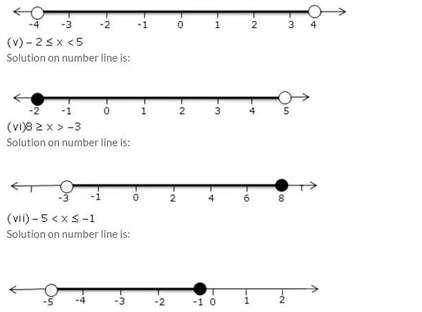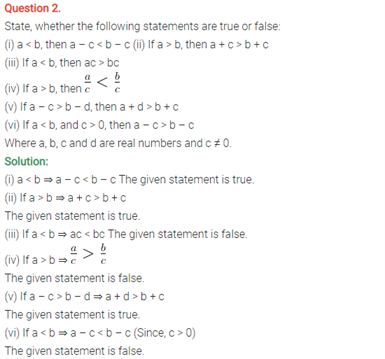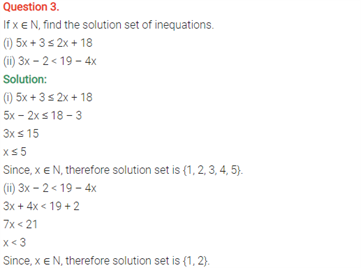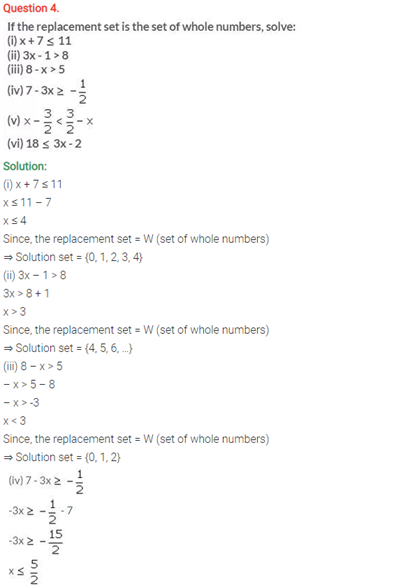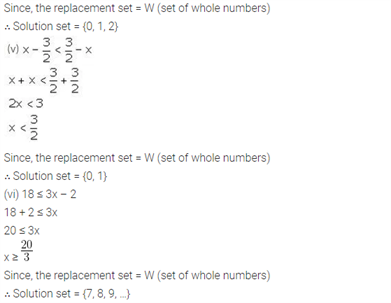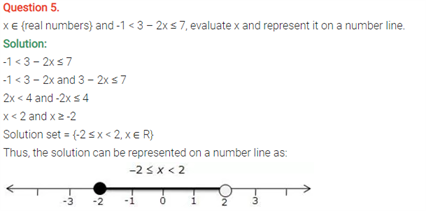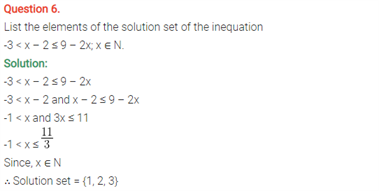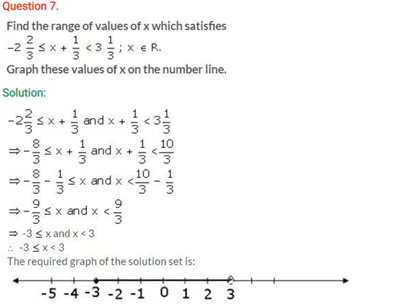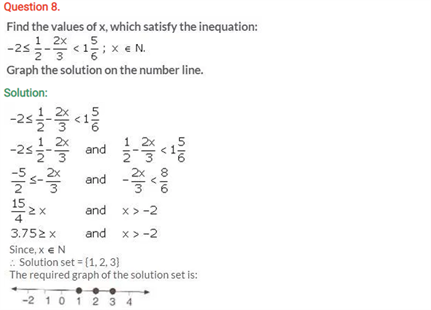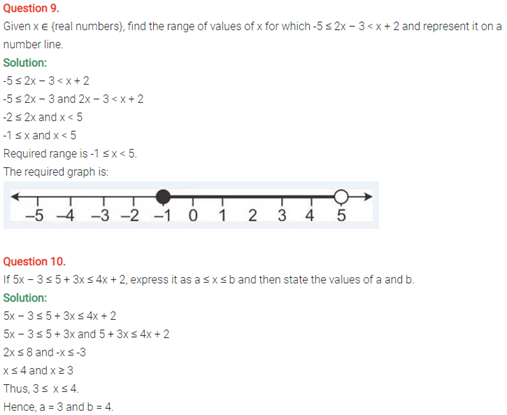# Selina Concise Maths Solution for ICSE Class 10 Chapter 4 Linear Inequations (In one variable)

## Selina Class 10 Maths Solutions for Chapter 4

Linear equations can be found in the ICSE Solutions For Class 10 Maths and are explained in a comprehensive manner. These questions will get you insights about linear equations and get a good grasp of this chapter. Lets us see the most basic definitions.

• A linear equation can be written in the form cx+d=e, where, c, d and e are real numbers and c not be equal to 0. Here the exponent of the variable is said to be one.
• Every linear equation has one true value of the variable or also known as a solution. However, not every linear equation with one variable has a single solution.
• There are two types of equations: contradiction and identities.

Students can find ICSE Class 10 Maths Selina Solutions Chapter 4 Linear Equations(In One Variable) below: